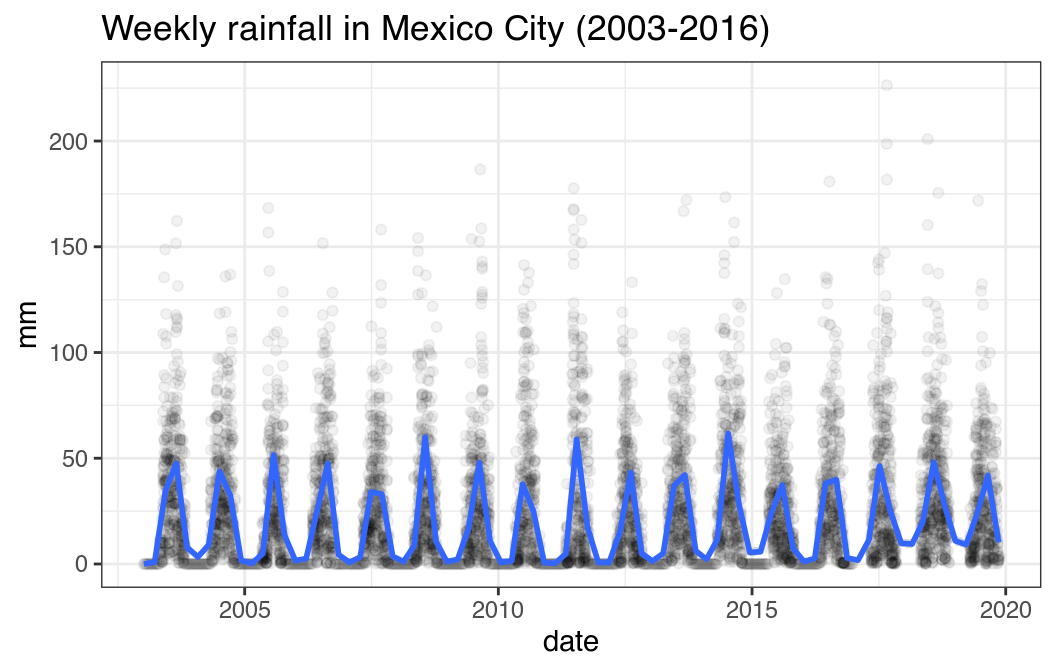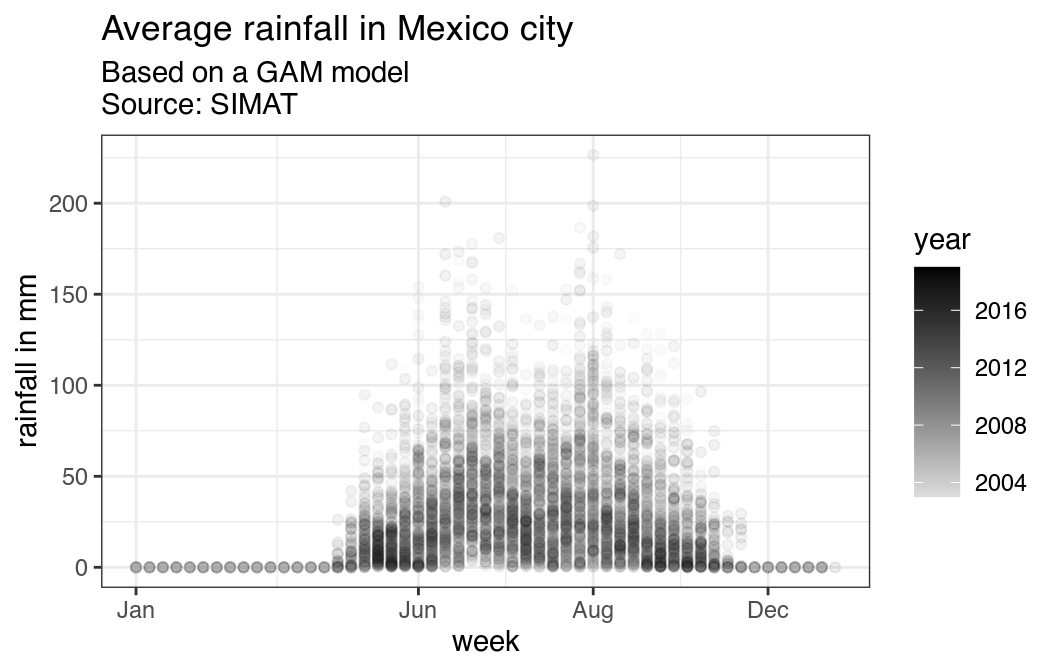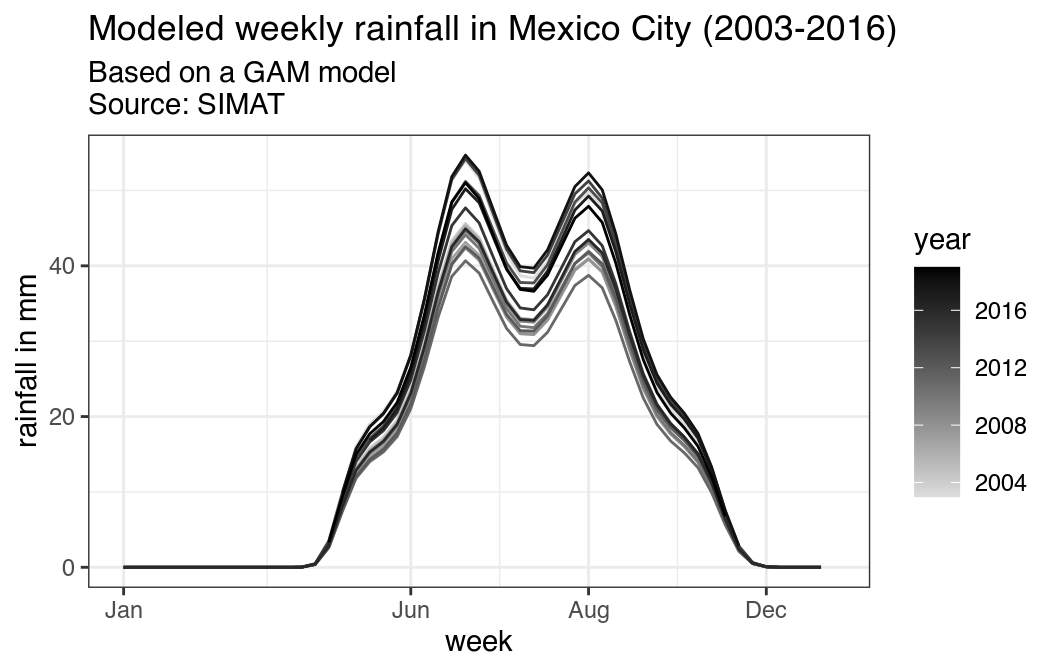## Auto-install required R packages
if (!require("pacman")) install.packages("pacman")
pacman::p_load(aire.zmvm, dplyr, ggplot2, lubridate, readr, stringr, mgcv)

df <- download_deposition(deposition = "HUMEDO", type = "CONCENTRACION") %>%
filter(pollutant == "PP")

# When does the SIMAT start recording rainfall each year?
knitr::kable(df %>%
group_by(year(date)) %>%
summarise(min = min(date)))
#> summarise() ungrouping output (override with .groups argument)
year(date) min
1997 1997-05-05
1998 1998-05-04
1999 1999-02-22
2000 2000-02-14
2001 2001-01-08
2002 2002-02-04
2003 2003-05-05
2004 2004-05-03
2005 2005-05-09
2006 2006-05-08
2007 2007-04-30
2008 2008-05-05
2009 2009-05-11
2010 2010-05-03
2011 2011-05-02
2012 2012-05-07
2013 2013-04-29
2014 2014-04-28
2015 2015-05-04
2016 2016-05-02
2017 2017-05-08
2018 2018-04-23
2019 2019-04-22

### Model with a GAM

# rainfall is measured around the begginig of may starting in May 2003
df <- df %>% filter(year(df$date) >= 2003) df$week <- week(df$date) df$year <- year(df$date) # no measures of rainfall outside the rainy season, so set them to zero df <- full_join(df, data.frame( year = year(seq(as.Date("2003-01-01"), as.Date("2016-12-31"), by = "week")), week = week(seq(as.Date("2003-01-01"), as.Date("2016-12-31"), by = "week")) ), by = c("year", "week")) %>% arrange(year, week) df$value[is.na(df$value)] <- 0 df$date <- as.Date(str_c(df$year, "-", df$week,"-",1), format = "%Y-%U-%u")
#fit <- gam(value ~ te(as.numeric(date), week, bs = c("tp", "cc"), k = c(10, 52)),
#           data = df, family = nb())
#plot(fit, pers = TRUE)
fit2 <- gam(value ~ s(as.numeric(date)) + s(week), data = df, family = nb())

summary(fit2)
#>
#> Family: Negative Binomial(1.361)
#>
#> Formula:
#> value ~ s(as.numeric(date)) + s(week)
#>
#> Parametric coefficients:
#>             Estimate Std. Error z value Pr(>|z|)
#> (Intercept)   1.3158     0.3952    3.33 0.000869 ***
#> ---
#> Signif. codes:  0 '***' 0.001 '**' 0.01 '*' 0.05 '.' 0.1 ' ' 1
#>
#> Approximate significance of smooth terms:
#>                       edf Ref.df  Chi.sq  p-value
#> s(as.numeric(date)) 8.336  8.876   66.32 4.15e-11 ***
#> s(week)             8.013  8.102 1717.86  < 2e-16 ***
#> ---
#> Signif. codes:  0 '***' 0.001 '**' 0.01 '*' 0.05 '.' 0.1 ' ' 1
#>
#> R-sq.(adj) =  0.186   Deviance explained = 34.7%
#> -REML =  27624  Scale est. = 1         n = 6556
df\$pred <- predict(fit2, newdata = df, type = "response")

### Plots

ggplot(df, aes(date, value)) +
geom_point(alpha = .05) +
geom_smooth(method = 'gam', formula = y ~ s(x, k = 53),
method.args= list(family = nb())) +
ggtitle("Weekly rainfall in Mexico City (2003-2016)") +
ylab("mm") +
theme_bw()ggplot(df, aes(week, value, group = year, color = year)) +
geom_point(alpha = .05) +
ggtitle("Average rainfall in Mexico city",
subtitle = "Based on a GAM model\nSource: SIMAT") +
ylab("rainfall in mm") +
scale_x_continuous(breaks = c(1,  week("2016-06-01"),
week("2016-09-01"), week("2016-12-01")),
labels = c("Jan", "Jun", "Aug", "Dec")) +
scale_color_continuous(low="#dddddd", high = "black") +
theme_bw()ggplot(df, aes(week, pred, group = year, color = year)) +
geom_line() +
ggtitle("Modeled weekly rainfall in Mexico City (2003-2016)",
subtitle = "Based on a GAM model\nSource: SIMAT") +
ylab("rainfall in mm") +
scale_x_continuous(breaks = c(1,  week("2016-06-01"),
week("2016-09-01"), week("2016-12-01")),
labels = c("Jan", "Jun", "Aug", "Dec")) +
scale_color_continuous(low="#dddddd", high = "black") +
theme_bw()